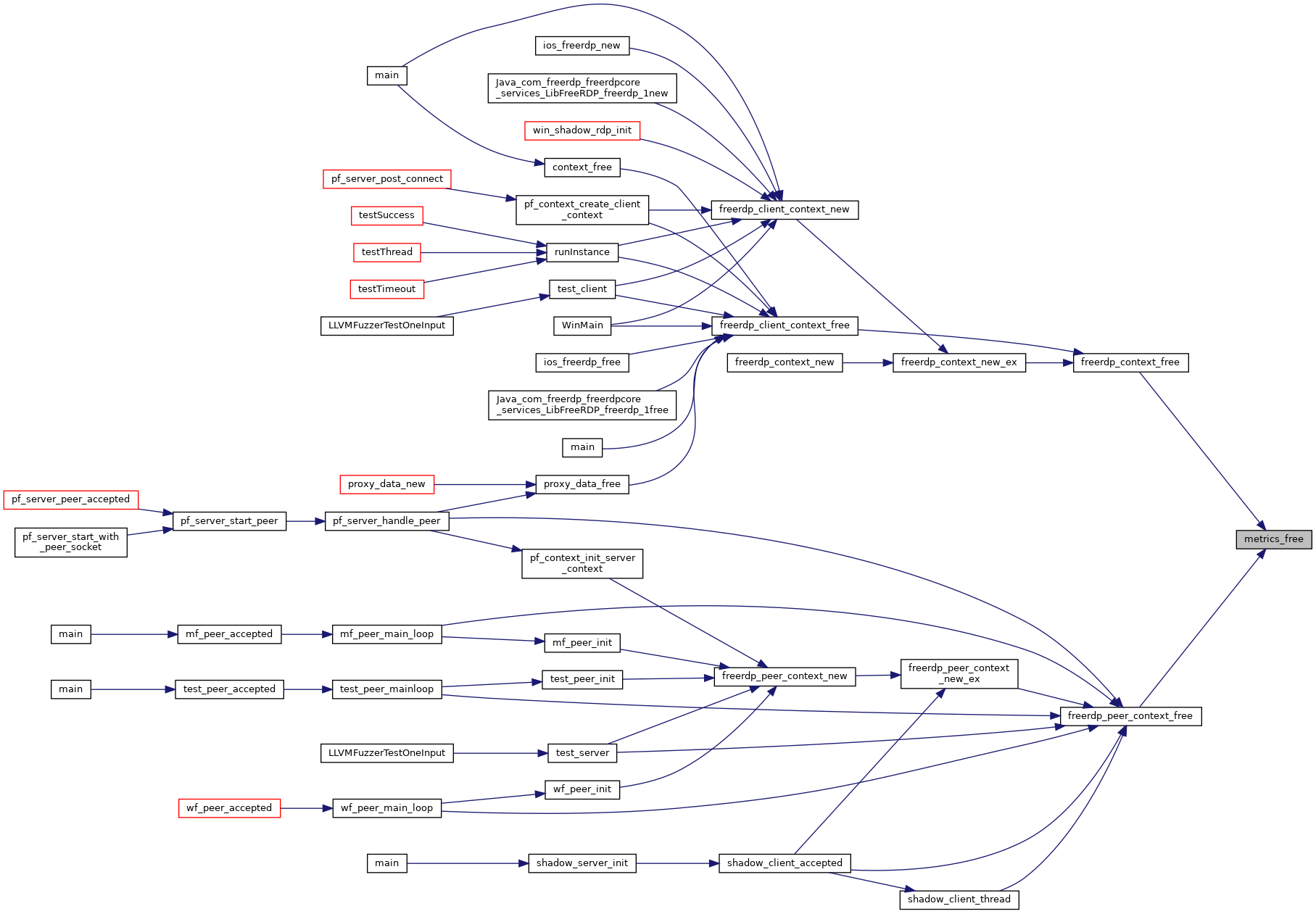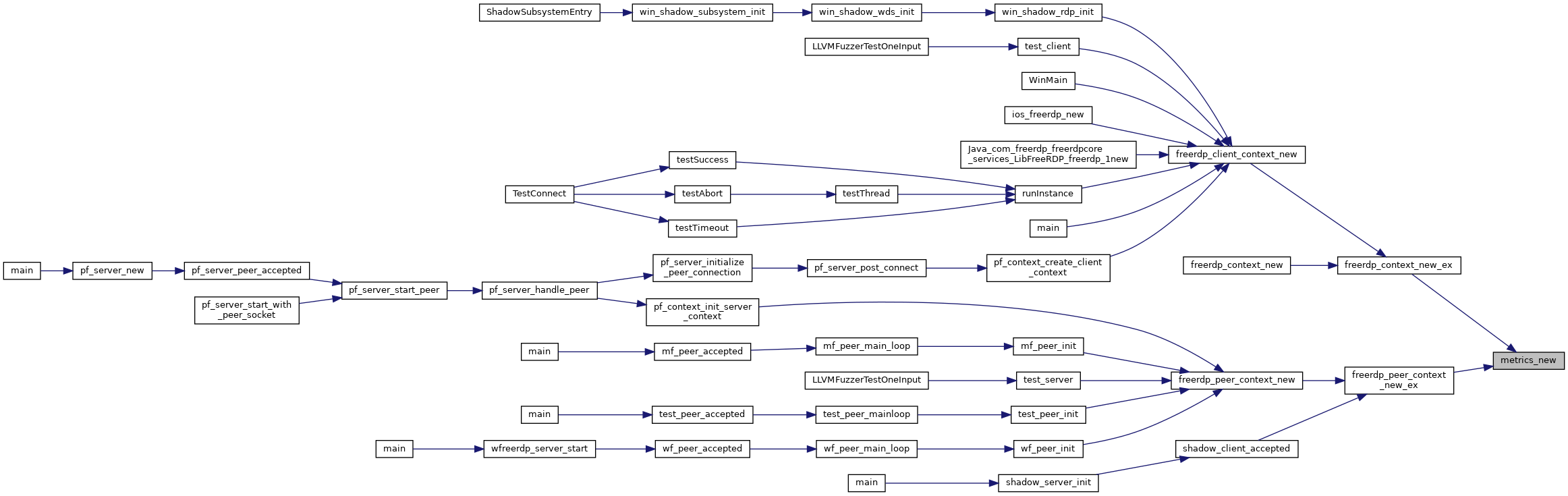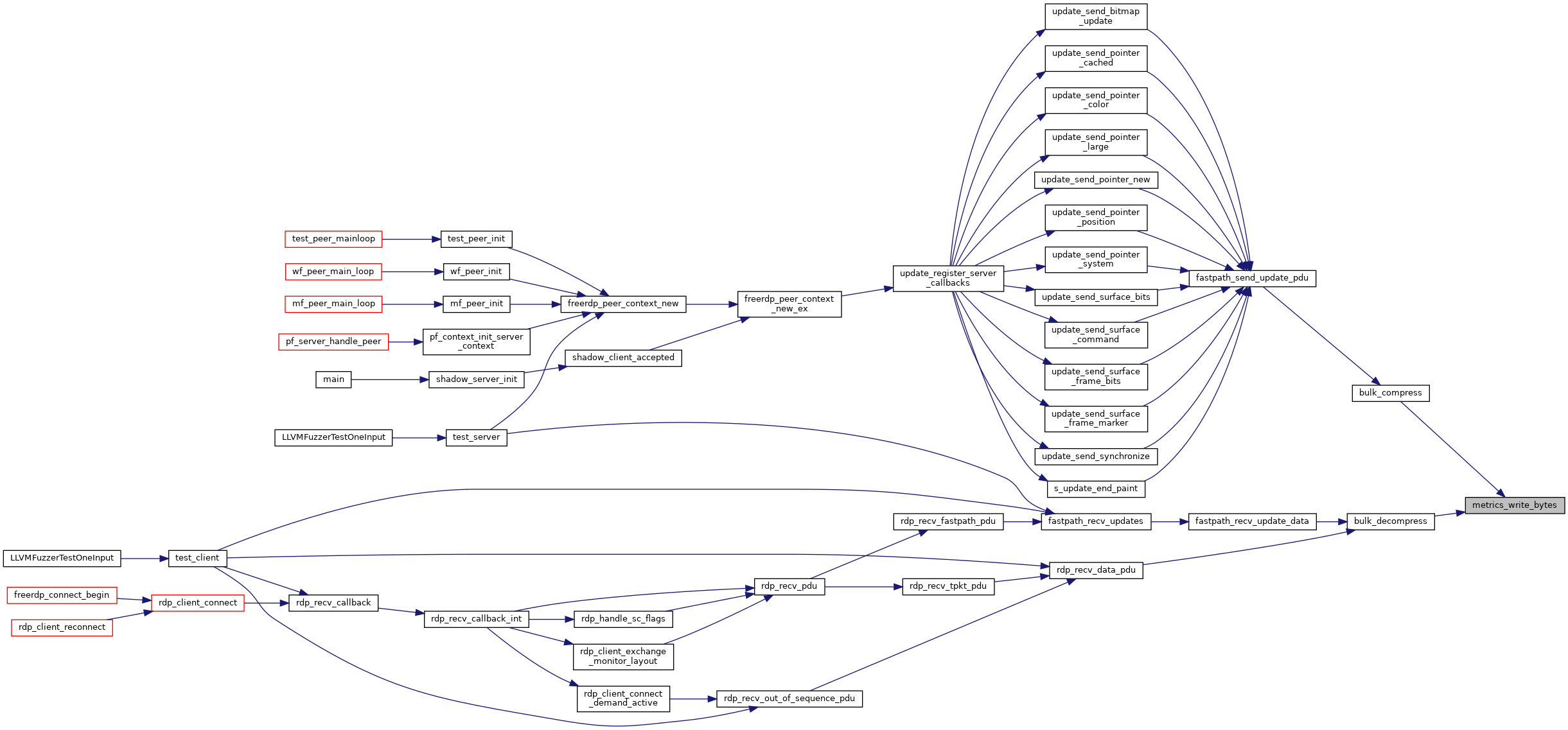FreeRDP
metrics.c File Reference
#include "rdp.h"

Functions

double metrics_write_bytes (rdpMetrics *metrics, UINT32 UncompressedBytes, UINT32 CompressedBytes)

rdpMetrics * metrics_new (rdpContext *context)

void metrics_free (rdpMetrics *metrics)

◆ metrics_free()

 void metrics_free ( rdpMetrics * metrics )
Here is the caller graph for this function:◆ metrics_new()

 rdpMetrics* metrics_new ( rdpContext * context )
Here is the caller graph for this function:◆ metrics_write_bytes()

 double metrics_write_bytes ( rdpMetrics * metrics, UINT32 UncompressedBytes, UINT32 CompressedBytes )

FreeRDP: A Remote Desktop Protocol Implementation Protocol Metrics